# Calculate the rotational inertia of a meter stick, with mass 0.342 kg, about an axis perpendicular...

Calculate the rotational inertia of a meter stick, with mass 0.342 kg, about an axis perpendicular to the stick and located at the 22.7 cm mark. (Treat the stick as a thin rod.)

This problem can be solved by applying the parallel axis theorem and noting that the rotational inertia about the midpoint of the stick and perpendicular to the stick is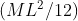where M is the mass of the stick and L is the length of the stick which is 1 meter here. The rotational inertia about the 22.7 cm mark comes out as 0.054 kg m^2. Detailed solution given below. If you have any doubt regarding the solution, ask me in the comment section. Feedback will be helpful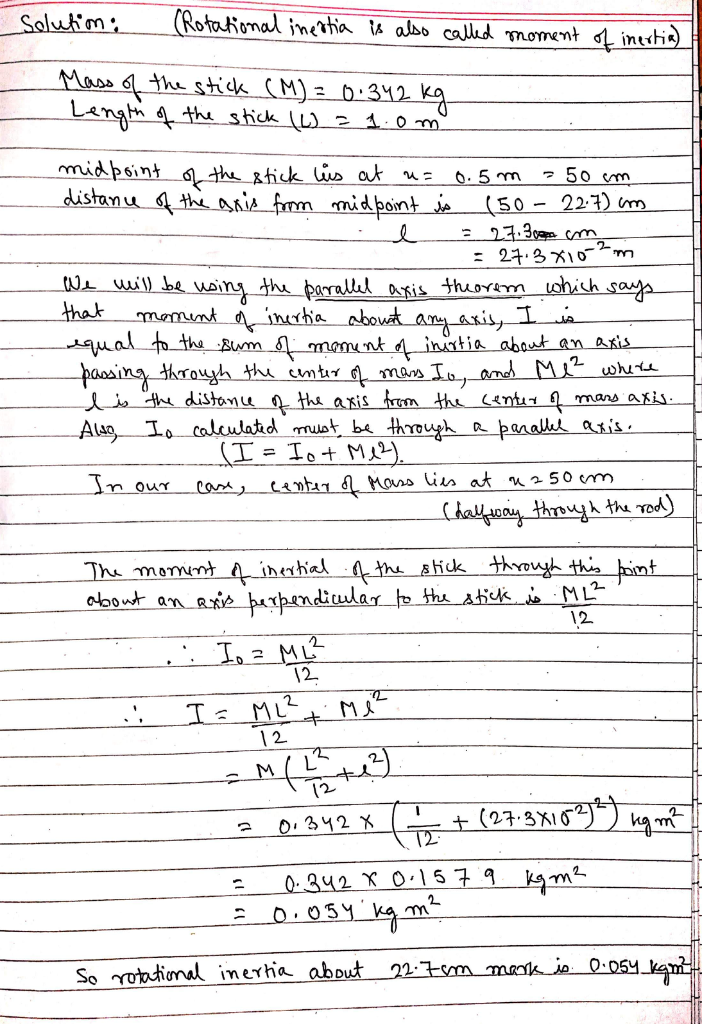#### Earn Coin

Coins can be redeemed for fabulous gifts.

Similar Homework Help Questions
• ### Calculate the rotational inertia of a meter stick, with mass 0.567 kg, about an axis perpendicular...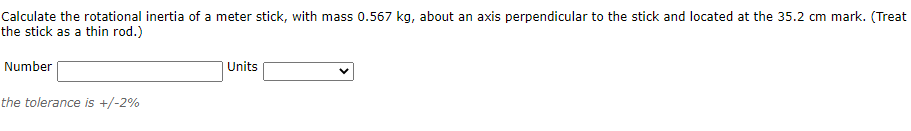Calculate the rotational inertia of a meter stick, with mass 0.567 kg, about an axis perpendicular to the stick and located at the 35.2 cm mark. (Treat the stick as a thin rod.) Number Units the tolerance is +/-2%

• ### Calculate the rotational inertia of a meter stick, with mass 0.58 kg, about an axis perpendicular to the stick and located at the 43 cm mark

Calculate the rotational inertia of a meter stick, with mass 0.58 kg, about an axis perpendicular to the stick and located at the 43 cm mark. (Treat the stick as a thin rod.) I know I = (1/12)(M)(L^2) and then I need to use the parallel-axis theorem so I need to add Mh^2 but that is not working. I get 0.156 doing this method but it is not correct.

• ### A particle of mass 0.500 kg is attached to the 100-cm mark of a meter stick...

A particle of mass 0.500 kg is attached to the 100-cm mark of a meter stick of mass 0.200 kg. The meter stick rotates on a frictionless, horizontal table with an angular speed of 2.00 rad/s. (a) Calculate the angular momentum of the system when the stick is pivoted about an axis perpendicular to the table through the 50.0-cm mark. (b) Calculate the angular momentum of the system when the stick is pivoted about an axis perpendicular to the table...

• ### A thin uniform rod (mass= 4.0 kg, length= 120.cm) rotates about an axis that is perpendicular...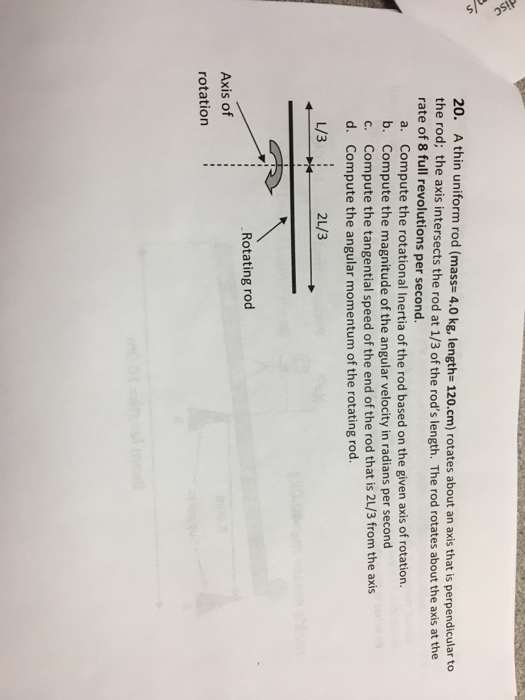A thin uniform rod (mass= 4.0 kg, length= 120.cm) rotates about an axis that is perpendicular to the rod; the axis intersects the rod at 1/3 of the rod's length. The rod rotates about the axis at the rate of 8 full revolutions per second. a. Compute the rotational Inertia of the rod based on the given axis of rotation. b. Compute the magnitude of the angular velocity in radians per second c. Compute the tangential speed of the end...

• ### PART A PART B PART C Q14 (10 points): If the rotational inertia about an axis...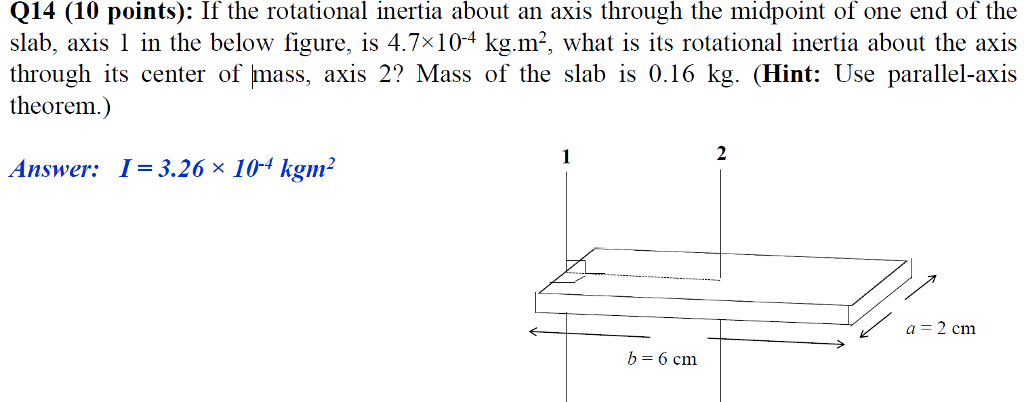PART A PART B PART C Q14 (10 points): If the rotational inertia about an axis through the midpoint of one end of the slab, axis 1 in the below figure, is 4.7x104 kg.m2, what is its rotational inertia about the axis through its center of mass, axis 2? Mass of the slab is 0.16 kg. (Hint: Use parallel-axis theorem.) Answer: I-3.26x I0 kgm2 a 2 cm -бст Q19 (10 points): A square slab of 0.4 m on each side...

• ### Exercise 6 Calculate the mass of a (real) meter stick using a pivot located at the...Exercise 6 Calculate the mass of a (real) meter stick using a pivot located at the 1 m 40 cm mark and a mass placed somewhere along the meter stick Movable Mass Information: such that the system is balanced (in equilibrium). Repeat the mass 1 50 g measurement with the pivot placed at the 30 cm mark. Enter both mass 2 40 g masses used (even if the same mass is used) and the locations on the Location 1 20...

• ### Determine the mass moment of inertia of the thin plate about the axis perpendicular to the...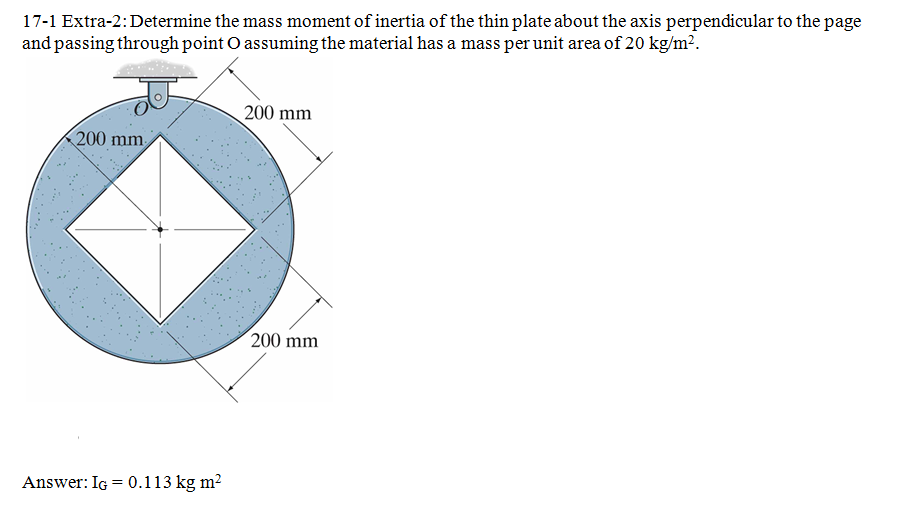Determine the mass moment of inertia of the thin plate about the axis perpendicular to the page and passing through point O assuming the material has a mass per unit area of 20 kg/m2.

• ### a uniform 172 g meter stick rotates about an axis perpendicular to the stick passing through...

a uniform 172 g meter stick rotates about an axis perpendicular to the stick passing through its center with an angular speed of 6.25 rad/s. (a) What is the magnitude of the angular momentum of the stick? (b) What would be the angular speed of the meter stick if it is rotated with the same angular momentum as in part (a) but about an axis of rotation situated at one end of the meter stick and parallel to the axis...

• ### (17%) Problem 2: A uniform wooden meter stick has a mass of m= 799 g. A...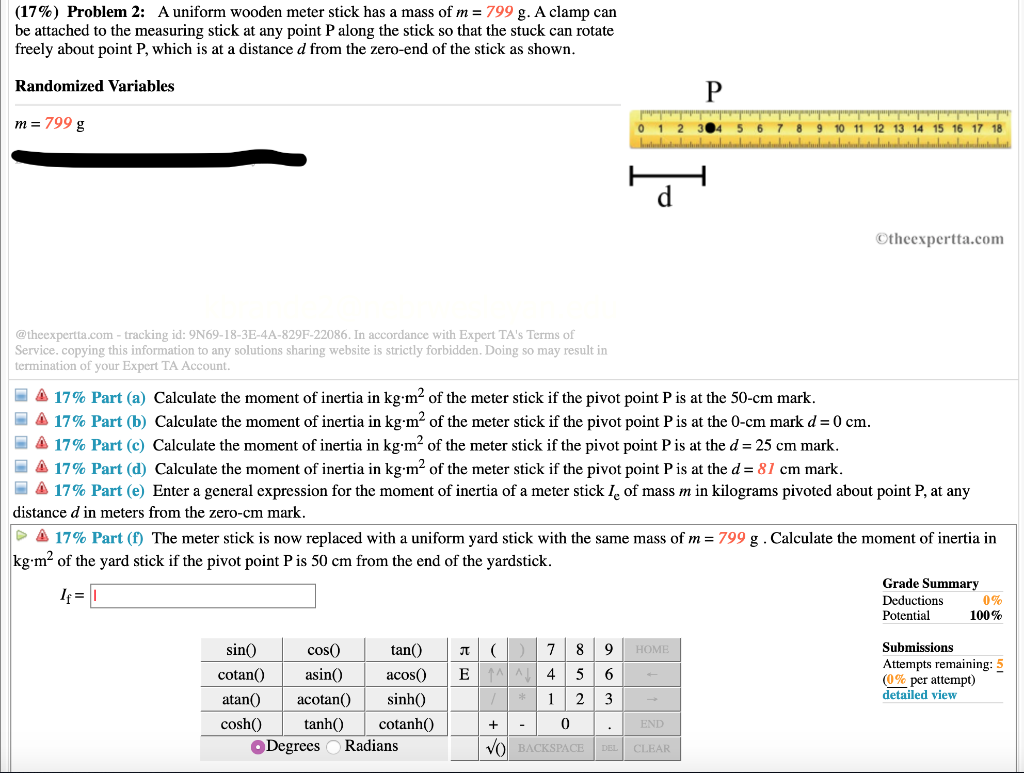(17%) Problem 2: A uniform wooden meter stick has a mass of m= 799 g. A clamp can be attached to the measuring stick at any point P along the stick so that the stuck can rotate freely about point P, which is at a distance d from the zero-end of the stick as shown. Randomized Variables m = 799 g 304 5 6 7 8 9 10 11 12 13 14 15 16 17 18 LLLLLLLL LLLLLLLL Otheexpertta.com @theexpertta.com...

• ### Procedure 1, 2, 3 Mass of a clamp: 21.5 True mass of meter stick: _133.6 g...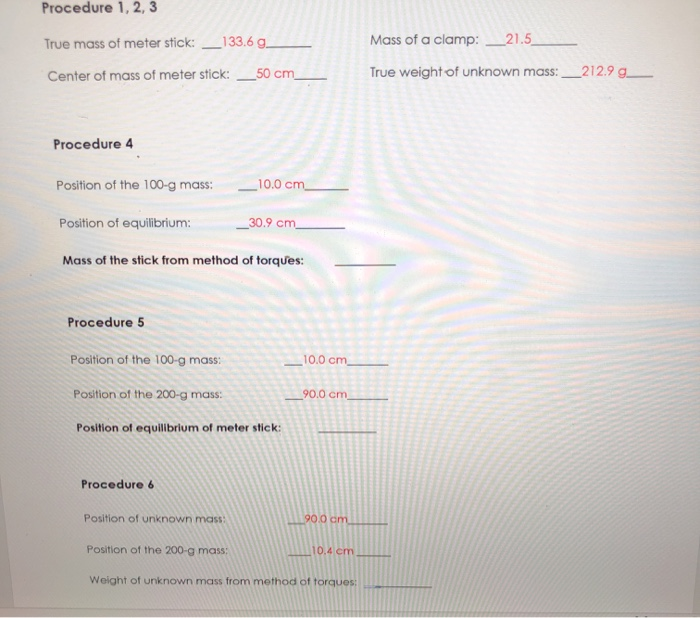Procedure 1, 2, 3 Mass of a clamp: 21.5 True mass of meter stick: _133.6 g Center of mass of meter stick: _50 cm True weight of unknown mass: _212.99_ Procedure 4 Position of the 100-g mass: 10.0 cm Position of equilibrium: _30.9 cm Mass of the stick from method of torques: Procedure 5 Position of the 100-g mass: 10.0 cm Position of the 200-g mass: _90.0 cm Position of equilibrium of meter stick: Procedure 6 Position of unknown mass...

Free Homework App